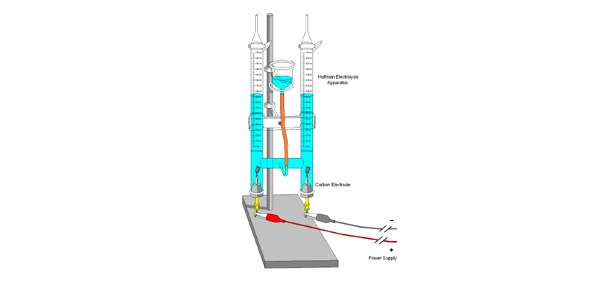# Metal And Electrolysis Test Review

20 Questions | Total Attempts: 53SettingsThe process of electrolysis is a technique wherein a direct electric current is utilised in order to achieve a chemical reaction that otherwise wouldn’t be expected. What can you tell us about how this process works? Take the quiz below and we’ll find out together!

Related Topics
• 1.
What is the main reducing agent in the reduction of iron ore?
• A.

Carbon monoxide

• B.

Slag

• C.

Carbon dioxide

• D.

Oxygen

• 2.
What are the main impurity in iron ore?
• A.

Steel

• B.

Rock

• C.

Silica

• D.

Limestone

• 3.
What is the definition of electrolysis?
• A.

The passage of a direct electric current through a solution containing ions

• B.

The use of an electric current to reduce iron ore

• C.

The process used to grow hair

• D.

Lightening

• 4.
What is the use of limestone in reducing iron ore?
• A.

Used to produce heat in blast furnace

• B.

It has no use

• C.

Reacts with the iron to make it explode

• D.

Added to the iron ore in a blast furnace, where it reacts with silica, and separates into iron and slag

• 5.
What is slag used for?
• A.

Pottery

• B.

Mixed with concrete to pave roads

• C.

Cereal

• D.

• 6.
Define Anion:
• A.

An ion with a positive charge that moves towards the negative electrode during electrolysis

• B.

An ion with a negative charge that moves towards the positive electrode during electrolysis

• C.

A positively charged electron by which electrons leave an electrical device

• D.

Electrolysis is the passage of a direct electric current through a solution containing ions.

• 7.
What is the charge of Potassium?
• A.

K++

• B.

K-

• C.

K+

• D.

K--

• 8.
What are the most commonly found iron ores?
• A.

Hematite and Magnetite

• B.

Silica and Limestone

• C.

Selamite and Hepatite

• D.

Hematite and Yellamite

• 9.
List the diatomic elements:
• A.

H, N, L, K, Cl, Fl, B

• B.

H, O, C, Mg, Cl, Ag, Na

• C.

H, N, O, F, Cl, I, Br

• D.

M, A, D, I, S, O, N,

• 10.
Define electrolyte:
• A.

A little electron

• B.

A negatively charged compound

• C.

A compound that in its molten or aqueous state conducts electricity

• D.

A special electrode

• 11.
True or False? A Cathode is a negatively charged electrode that is the source of electrons entering an electronic device.
• A.

False

• B.

True

• 12.
What would be the product of electrolysis in the following compound:  PbBr2 (s)
• A.

It remains a solid

• B.

Pb (l) 2Br (g)

• C.

Pb (g) Br (l)

• D.

It does not react

• 13.
How is Coke produced?
• A.

Mixing bubbles and flavoring

• B.

Heating limestone in a blast furnace

• C.

In the extraction of iron ore

• D.

Heating coal in the absence of air

• 14.
True or false? A cation is an ion with a positive charge that moves towards the negative electrode during electrolysis.
• A.

True

• B.

False

• 15.
How much of the Earth's crust is made of iron?
• A.

5.6%

• B.

20.3%

• C.

0%

• D.

50.5%

• 16.
What is stainless steel used for?
• A.

Phones, books

• B.

Cutlery, cooking utensils, kitchen sinks

• C.

Computers, tables, bridges

• D.

Walls

• 17.
Silica is in what state at room temperature?
• A.

Solid

• B.

Liquid

• C.

Gas

• D.

England

• 18.
What is used industrially to reduce iron ore?
• A.

A fire pit

• B.

A blast furnace

• C.

An oven

• D.

Microwave

• 19.
Carbon dioxide reacts with coke to form?
• A.

They don't react

• B.

Silica

• C.

Carbon dioxide

• D.

Carbon monoxide

• 20.
Did you enjoy this quiz?
• A.

Yes!

• B.

No.

• C.

I love chemistry

• D.

Sure?